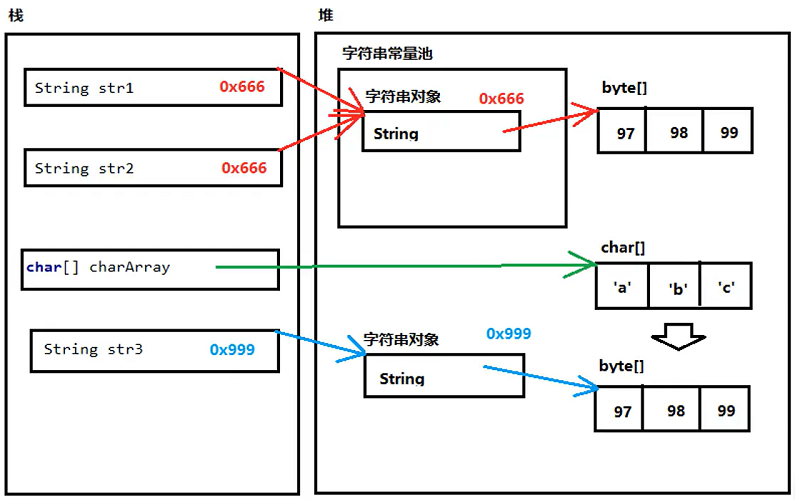### 一、CharSequence

CharSequence是一个描述字符串结构的接口，在这个接口里面一般发现有三种常用的子类：

• Stirng类
• StringBuffer类
• StringBuilder类

### 二、String

java.lang.String 类代表字符串。Java 程序中的所有字符串文字（例如 `"abc"` ）都被实现为此类的实例。特点：

• 字符串的值在创建后不能被更改；
• 因为 String 对象是不可变的，它们是可以被共享；
• jdk1.8 的 String 使用的是 char 数组。

#### 1、创建字符串的方法

直接创建。

``````1String str = "zyxwmj.top";
``````

初始化新创建的 String对象，使其表示空字符序列。

``````1String()
``````

根据字节数组创建字符串。

• offset 和 length 为可选：offset 为起始下标，length 为长度，offset 和 length 必须同时存在。
• charset 和 charsetName 为可选：使用该 charset 对 bytes 进行解码，两个参数只能存在一个。
``````1String(byte[] bytes, int offset, int length, Charset charset)
2String(byte[] bytes, int offset, int length, String charsetName)
``````

根据字符数组创建字符串。

``````1String(char[] value, int offset, int count)
``````

#### 2、字符串的比较

##### （1）==

字符串常量池，程序当中直接写上的双引号字符串，就在字符串常量池中。

• 对于基本类型来说，== 是进行数值的比较。
• 对于引用类型来说，== 是进行地址值的比较。
`````` 1class Solution {
2    public static void main(String[] args) {
3        String str1 = "zyx";
4        String str2 = "zyx";
5        char[] chars = new char[]{'z', 'y', 'x'};
6        String str3 = new String(chars);
7
8        System.out.println(str1 == str2);// true
9        System.out.println(str1 == str3);// false
10        System.out.println(str2 == str3);// false
11    }
12}
``````

双引号直接写的字符串在常量池当中，new 的不在池中。##### （2）是否相等

将两个字符串按值比较。

``````1boolean equals(Object anObject)
``````

将两个字符串按值比较，不区分大小写。

``````1boolean equalsIgnoreCase(String anotherString)
``````
`````` 1class Solution {
2    public static void main(String[] args) {
3        String str1 = "zyx";
4        String str2 = "ZYX";
5        char[] chars = new char[]{'z', 'y', 'x'};
6        String str3 = new String(chars);
7
8        System.out.println(str1.equals(str2));// false
9        System.out.println(str1.equals(str3));// true
10        System.out.println(str1.equalsIgnoreCase(str2));// true
11    }
12}
``````

当前字符串与指定的 CharSequence 进行比较。

``````1boolean contentEquals(CharSequence cs)
``````

当前字符串与指定的 StringBuffer 进行比较。

``````1boolean contentEquals(StringBuffer sb)
``````
##### （3）比较字符串返回 int

按字典顺序比较两个字符串，相等则为 0，小于 0 则表示当前字符串比比较的字符串小，否则大。

``````1int compareTo(String anotherString)
``````

按字典顺序比较两个字符串，忽略大小写。

``````1int compareToIgnoreCase(String str)
``````
##### （4）指定区域是否相等

判断两个区域的字符串是否相等。toffset 表示当前字符串的起始偏移量；other 为字符串参数；ooffset 为比较字符串的起始偏移量；len 为要比较的字符数。

``````1boolean regionMatches(int toffset, String other, int ooffset, int len)
``````

ignoreCase 为 true 表示不区分大小写。

``````1boolean regionMatches(boolean ignoreCase, int toffset, String other, int ooffset, int len)
``````

#### 3、字符串匹配

##### （1）是否包含指定字符串

当前字符串包含指定字符串时，返回true。

``````1boolean contains(CharSequence s)
``````
##### （2）前后缀是否为指定字符串

判断当前字符串是否以指定的后缀结尾。

``````1boolean endsWith(String suffix)
``````

判断当前字符串是否以指定的前缀开头。

``````1boolean startsWith(String prefix)
``````

判断当前字符串在指定索引处开始的子字符串中是否以指定的前缀开头。

``````1boolean startsWith(String prefix, int toffset)
``````
##### （3）字符串在当前字符串第一次出现的索引

返回指定字符第一次出现的字符串内的索引。

``````1int indexOf(int ch)
``````

返回指定字符第一次出现的字符串内的索引，以指定的索引开始搜索。

``````1int indexOf(int ch, int fromIndex)
``````

返回指定子字符串第一次出现的字符串内的索引。

``````1int indexOf(String str)
``````

返回指定子串的第一次出现的字符串中的索引，从指定的索引开始。

``````1int indexOf(String str, int fromIndex)
``````
##### （4）字符串在当前字符串最后一次出现的索引

返回指定字符的最后一次出现的字符串中的索引。

``````1int lastIndexOf(int ch)
``````

返回指定字符的最后一次出现的字符串中的索引，从指定的索引开始向后搜索。

``````1int lastIndexOf(int ch, int fromIndex)
``````

返回指定子字符串最后一次出现的字符串中的索引。

``````1int lastIndexOf(String str)
``````

返回指定子字符串的最后一次出现的字符串中的索引，从指定索引开始向后搜索。

``````1int lastIndexOf(String str, int fromIndex)
``````
##### （5）正则表达式

判断当前字符串是否匹配指定正则表达式。

``````1boolean matches(String regex)
``````

#### 4、字符串的分割与替换

##### （1）字符串的分割

返回一个下标为 [beginIndex, length-1] 的字符串。

``````1String substring(int beginIndex)
``````

返回一个下标为 [beginIndex, endIndex-1] 的字符串。

``````1String substring(int beginIndex, int endIndex)
``````

按照指定的正则表达式对字符串进行分割。`.``\$``|``*` 等转义字符，必须得加 `\\`

``````1String[] split(String regex)
``````

limit 表示分割的份数。

``````1String[] split(String regex, int limit)
``````
##### （2）字符串的替换

将原字符串中的所有 oldChar 替换为 newChar。

``````1String replace(char oldChar, char newChar)
``````

将原字符串中的所有 target 替换为 replacement。

``````1String replace(CharSequence target, CharSequence replacement)
``````

将原字符串中的所有 regex 替换为 replacement。

``````1String replaceAll(String regex, String replacement)
``````

将原字符串中的第一个 regex 替换为 replacement。

``````1String replaceFirst(String regex, String replacement)
``````

#### 5、返回指定格式的字符串

##### （1）返回当前字符串
``````1String intern()
``````
• 直接使用双引号声明出来的 String 对象会直接存储在常量池中。
• 如果不是用双引号声明的 String 对象，可以使用 String 提供的 intern 方法。intern 方法会从字符串常量池中查询当前字符串是否存在，若不存在就会将当前字符串放入常量池中，再返回。
``````1class Solution {
2    public static void main(String[] args) {
3        String str1 = new String("zyx");
4        String str2 = "zyx";
5        System.out.println(str1.intern() == str2); // ture
6        System.out.println(str1 == str2); // false
7    }
8}
``````
##### （2）生成新的字符串

返回一个新的字符串，每个 elements 用 delimiter 分隔。

``````1static String join(CharSequence delimiter, CharSequence... elements)
``````

返回一个新的字符串，每个 elements 用 delimiter 分隔。

``````1static String join(CharSequence delimiter, Iterable<? extends CharSequence> elements)
``````
`````` 1class Solution {
2    public static void main(String[] args) {
3        String str1 = String.join("-", "Java", "is", "cool");
4        System.out.println(str1);// Java-is-cool
5
6        List<String> list = new LinkedList<>();
10        String str2 = String.join(" ", list);
11        System.out.println(str2); // Java is cool
12    }
13}
``````
##### （3）更改字母的大小写

将此字符串使用默认语言环境的规则，转为小写。

``````1String toLowerCase()
``````

将此字符串转使用给定的规则，转为小写 Locale 。

``````1String toLowerCase(Locale locale)
``````

将此字符串使用默认语言环境的规则，转为大写。

``````1String toUpperCase()
``````

将此字符串转使用给定的规则，转为大写 Locale 。

``````1String toUpperCase(Locale locale)
``````
##### （4）字符串拼接

拼接字符串返回新的字符串。

``````1String concat(String str)
``````
##### （5）去掉字符串的首尾空格

返回一个字符串，并删除任何前导和尾随空格。

``````1String trim()
``````
##### （6）将字符串返回成指定格式

返回 char指定索引处的值。

``````1char charAt(int index)
``````

返回指定索引处的字符（Unicode代码点），即 byte。

``````1int codePointAt(int index)
``````

获取当前字符串的字符数组。

``````1byte[] getBytes()
``````

将此字符串转换为新的字符数组。

``````1char[] toCharArray()
``````

将当前字符串从 srcBegin 到 srcEnd-1 位置上的字符复制到字符数组 dst 中，并从 dst 的 dstBegin 处开始存放。

``````1void getChars(int srcBegin, int srcEnd, char[] dst, int dstBegin)
``````

将其他类型转换为字符串。

``````1static String valueOf(Object obj)
``````

### 三、StringBuffer 和 StringBuilder

StringBuilder 是线程不安全的，StringBuffer 是线程安全的。

#### 1、追加和插入字符

使用 append 可以向字符串末尾追加 Object 、指定长度的 char[] 和 指定长度的字符串，但不能直接追加 byte[] 数组。

``````1StringBuffer append(char[] str, int offset, int len)
2StringBuffer append(CharSequence s, int start, int end)
3StringBuffer append(Object obj)
``````

添加 byte 使用 appendCodePoint。

``````1StringBuffer appendCodePoint(int codePoint)
``````

插入和追加类似，只需指定加入的下标即可。

``````1StringBuffer insert(int offset, Object obj)
``````

#### 2、删除和修改字符

删除下标为 [start ~ end-1] 的字符。

``````1StringBuffer delete(int start, int end)
``````

删除下标为 index 的字符。

``````1StringBuffer deleteCharAt(int index)
``````

修改下标为 index 的字符。

``````1void setCharAt(int index, char ch)
``````

#### 3、其他方法

返回当前容量。

``````1int capacity()
``````

字符串反转。

``````1StringBuffer reverse()
``````

设置字符序列的长度，溢出的字符删除。如果 `newLength <= capacity()` 则容量不变，否则 `capacity() = capacity() * 2 + 2`

``````1void setLength(int newLength)
``````

确保容量至少等于规定的最小值。如果 `minimumCapacity <= capacity()` 则容量不变，否则 `capacity() = capacity() * 2 + 2`

``````1void ensureCapacity(int minimumCapacity)
``````

尝试减少用于字符序列的存储，将 `capacity()` 设置为字符串有效长度。

``````1void trimToSize()
``````

返回一个下标为 [start,end-1] 的字符串，和 substring 的返回类型不同。

``````1CharSequence subSequence(int start, int end)
``````